3rd of September, 2008

1.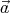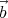, so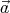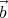= 0 and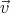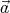= α. Multiplying the first equation byfrom the left (using the cross product), we get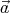× (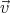×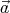) =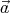×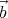a2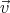-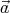(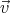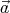) =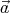×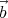. Using that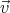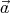= α,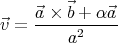1.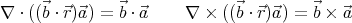2.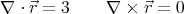3.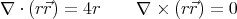(Note: The curl of any radially symmetric vector field is 0.)

4.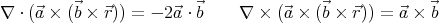5.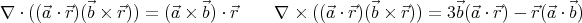6.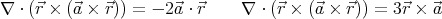The curl of an electrostatic field is 0, so if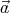and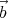are not parallel, then only the fields in points (b) and (c) may be electrostatic fields.

1. The charge density is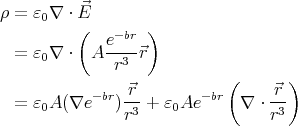Let us calculate the two terms of this sum separately: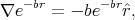where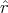=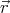∕r, and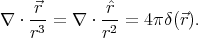Thus the complete charge density is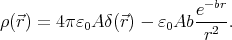2. The total charge enclosed by a spherical surface S of radius r centred around the origin is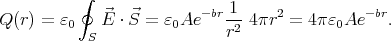Thus as r →∞, the total charge enclosed by the surface goes to 0.

2. The work needed to add another layer of charge dq to a sphere of radius r and total charge q is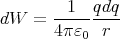The sphere has a homogeneous charge distribution, so q =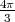r3ρ and dq = 4πρr2dr.

Thus the energy needed to assemble a charged sphere of radius R and charge Q is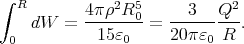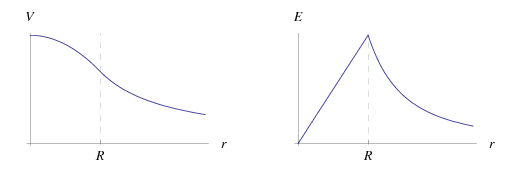Figure 1: The potential and electric field as a function of the distance from the centre of the sphere.

The electric field is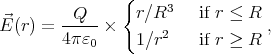and the potential is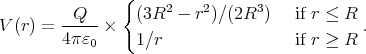3. Let us find the gravitational potential energy W(x) of the two half-spheres as a function of their separation x. The force keeping them together is simply F = dW∕dx.

Creating a gap by separating the halves is equivalent to removing (“mining out”) all the matter from the gap, and distributing it on the surface (i.e. it causes the same change ΔW in energy). Provided that the gap between the halves is very narrow compared to the planet’s radius (x R), the change in the gravitational field is negligible.

Using Gauß’s law, it is easily shown that the gravitational potential below the surface of the spherical planet is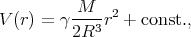where γ is the gravitational constant, M is the mass of the planet, and r is the distance from the centre.

When moving a piece of mass dm up to the surface, the increase in energy isV (R) - V (r)dm. To get the total change in energy, we must integrate over the volume of the gap: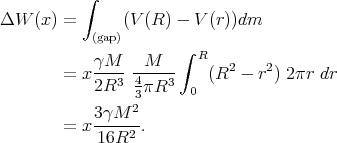(1)

The force keeping the halves together is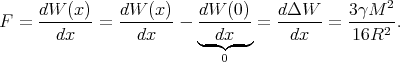(I.e. the same as the weight of an object of mass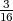M on the surface of the planetoid.)

Back to the main page